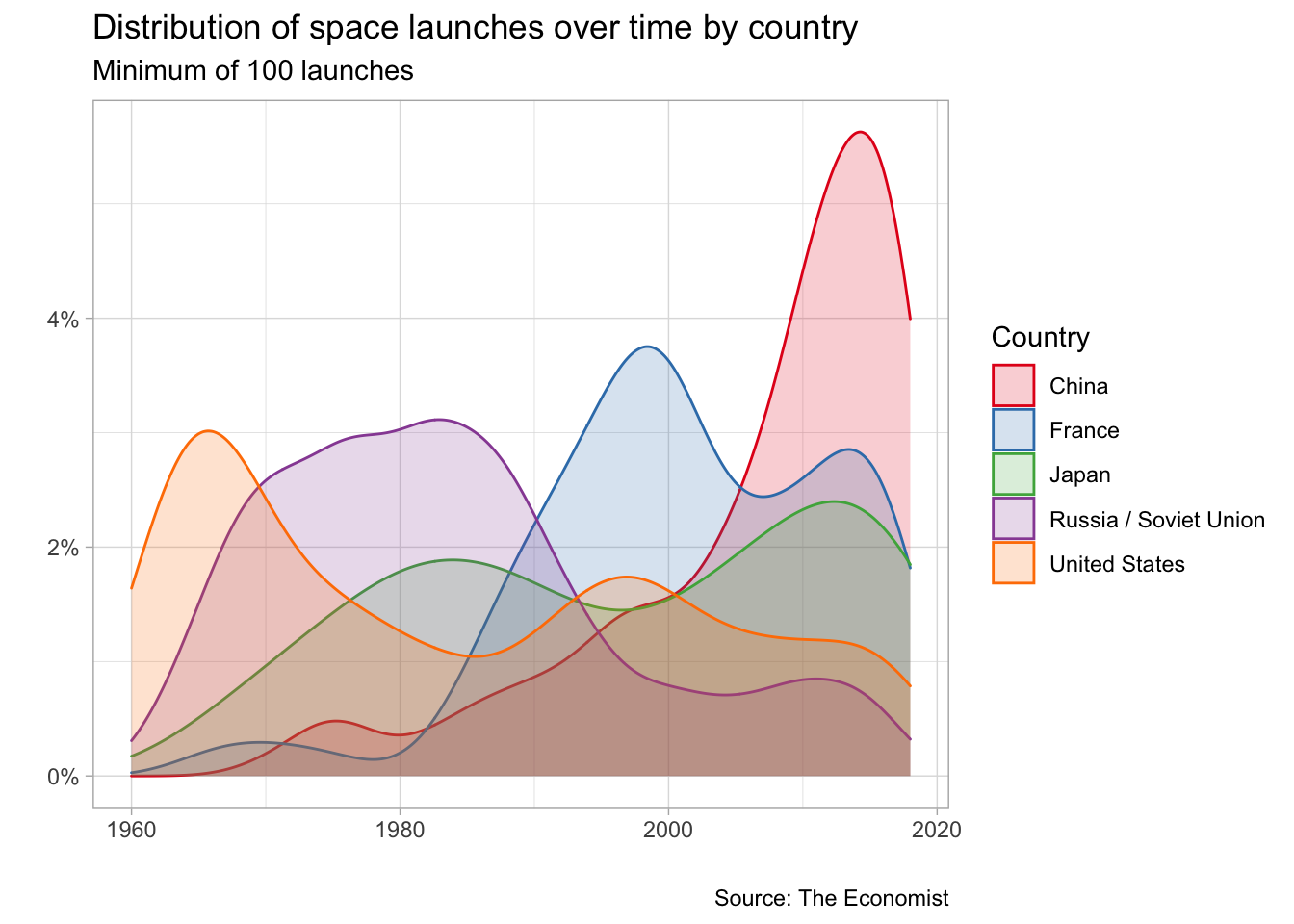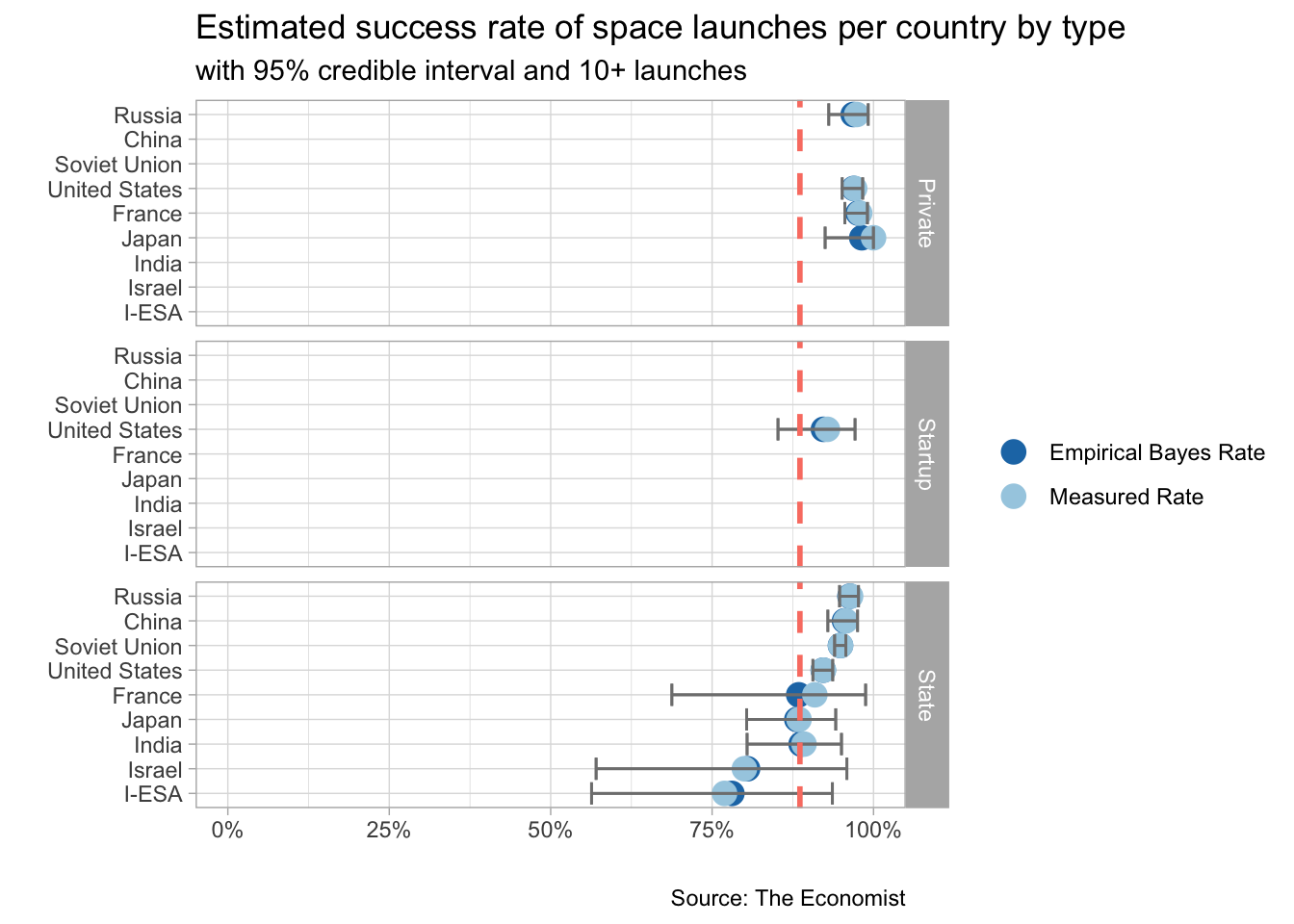Analyzing data for #tidytuesday week of 1/15/2019 (source)

``````# LOAD PACKAGES AND PARSE DATA
library(tidyverse)
library(RColorBrewer)
library(forcats)
library(scales)
library(ebbr)

launches <- launches_raw %>%
filter(launch_year >= '1960')``````

### Distribution of the most space launches over time?

``````countries <- launches %>%
count(state_code, sort = TRUE) %>%
filter(n >= 100)

launches %>%
inner_join(countries) %>%
# INCOMING NASTY IFELSE CODE (NEED TO REFACTOR)
mutate(state_code = ifelse(state_code == 'RU', 'Russia / Soviet Union',
ifelse(state_code == 'SU', 'Russia / Soviet Union',
ifelse(state_code == 'US', 'United States',
ifelse(state_code == 'CN', 'China',
ifelse(state_code == 'IN', 'India',
ifelse(state_code == 'F', 'France',
ifelse(state_code == 'J', 'Japan', state_code)))))))) %>%
ggplot() +
geom_density(aes(launch_year, fill = state_code, color = state_code),
alpha = 0.2) +
theme_light() +
scale_color_brewer(palette = 'Set1') +
scale_fill_brewer(palette = 'Set1') +
labs(x = "",
y = "",
title = "Distribution of space launches over time by country",
subtitle = "Minimum of 100 launches",
caption = "Source: The Economist",
fill = "Country",
color = "Country") +
scale_y_continuous(labels = percent_format(round(1)))``````### Who has a better success rate: private, startup or states ?

``````launches %>%
mutate(category = ifelse(category == 'O', 1, 0)) %>%
select(launch_year, agency_type, category) %>%
group_by(launch_year, agency_type) %>%
summarize(success = sum(category),
total = n(),
rate = success / total) %>%
ungroup() %>%
mutate(agency_type = str_to_title(agency_type)) %>%
ggplot() +
geom_line(aes(launch_year, .fitted, color = agency_type),
size = 1) +
geom_ribbon(aes(x = launch_year, ymin = .low, ymax = .high, fill = agency_type),
alpha = 0.1) +
theme_light() +
scale_fill_brewer(palette = 'Set1') +
scale_color_brewer(palette = 'Set1') +
labs(x = "",
y = "",
caption = "Source: The Economist",
title = "Success rate of space launches by type",
subtitle = "Empirical Bayes rate @ 95% credible interval",
color = "Type",
fill = "Type") +
scale_y_continuous(labels = percent_format(round(1)),
limits = c(0,1))``````
``````## Warning: `data_frame()` is deprecated as of tibble 1.1.0.
## This warning is displayed once every 8 hours.
## Call `lifecycle::last_warnings()` to see where this warning was generated.``````### Success rate for each country by agency type ?

``````# APPLY EMPIRICAL BAYESIAN STATS TO DATASET
launches_parsed <- launches %>%
mutate(category = ifelse(category == 'O', 1, 0),
agency_type = str_to_title(agency_type)) %>%
select(launch_year, state_code, agency_type, category) %>%
group_by(state_code, agency_type) %>%
summarize(success = sum(category),
total = n(),
rate = success / total) %>%
ungroup() %>%

# PLOT THE GRAPH
launches_parsed %>%
filter(total >= 10) %>%
select("Empirical Bayes Rate"=.fitted,
"Measured Rate"=.raw,
everything()) %>%
gather(key, value, `Empirical Bayes Rate`:`Measured Rate`) %>%
# INCOMING NASTY IFELSE CODE (NEED TO REFACTOR)
mutate(state_code = ifelse(state_code == 'RU', 'Russia',
ifelse(state_code == 'SU', 'Soviet Union',
ifelse(state_code == 'US', 'United States',
ifelse(state_code == 'CN', 'China',
ifelse(state_code == 'IN', 'India',
ifelse(state_code == 'F', 'France',
ifelse(state_code == 'J', 'Japan',
ifelse(state_code == 'IL', 'Israel', state_code))))))))) %>%
ggplot() +
geom_point(aes(x=reorder(state_code, value), y=value, color = key), size = 4) +
geom_errorbar(aes(x = state_code, ymin = .low, ymax = .high), size = 0.5, color = "gray50") +
geom_hline(data=launches_parsed, aes(yintercept = median(.fitted)), color = 'salmon', linetype = 'dashed', size = 1) +
coord_flip() +
theme_light() +
scale_y_continuous(labels = percent_format(round(1)),
limits = c(0,1)) +
labs(x = "",
title = "Estimated success rate of space launches per country by type",
subtitle = "with 95% credible interval and 10+ launches",
y = "",
caption = "Source: The Economist",
color = "") +
scale_color_brewer(palette = 'Paired', direction = -1) +
facet_grid(agency_type~.)``````